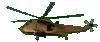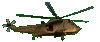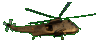Perfect Trinomial Square    Factoring      Fun Help     Game Tips:

- Perfect squares are formed by multiplying a quantity times itself, as in (3)(3) =9 .
Here are six perfect squares: 16,  25,  (-6)2,  r2,  (y+3)2,   (2w-5t)2 .

- A 'Perfect Trinomial Square' is the square of a binomial.
For example, the perfect trinomial a2+2ab+b2 can be formed from (a+b)2.
This means a2+2ab+b2 can be factored as (a+b)(a+b).

- Similarly, the 'Perfect Trinomial Square'  a2-2ab+b2  can be factored into the two factors (a-b)(a-b).
If you check (a-b)(a-b) , it multiplies together to give you the perfect trinomial a2-2ab+b2.
[To review multiplying a binomial times a binomial see the other SliderMath games.]

- The 'Perfect Trinomial Square'  y2+6y+9  can be factored as (y+3)(y+3).
- The 'Perfect Trinomial Square'  4w2-20wt+25t2  can be factored as (2w-5t)(2w-5t).

- Your Game Score is reduced by the number of helicopter hits.

- To slow the game speed repeat tap/click on the word Slider.
- To increase the game speed repeat tap/click on the word Math.
- Speed can also be adjusted with a keyboard's - and + keys.

- Refresh/Reload the web page to restart the game.